## 1.1 Introduction.

Pressure vessels are used to hold compressed gas. As per gas cylinder rules 2004 published by the gazette of India, “Compressed gas” means any permanent gas, liquefiable gas or gas dissolved in liquid under pressure or gas mixture which in a closed gas cylinder exercises a pressure either exceeding 2.5MPa at +15°C or a pressure exceeding 3MPa at +50°C or both. Pressure vessels are found in many mechanical systems such as chemical plants, airplanes, ships, submersible vehicles and manufacturing systems. The most common forms of pressure vessels to hold liquids or gases under internal pressure are spheres and closed end cylinders. The commonly used pressure vessel is the domestic gas cylinder.

## 1.2 Background.

### 1.2.1 Size and pressure.Figure 1.1 Dimensions of domestic gas cylinder. [1 &2]
Figure 2.1 shows a domestic LPG cylinder. The density of the LPG is approximately half that of water and ranges from 525 to 580 kg /m3 @ 15°C. If we consider a domestic gas cylinder, it will be filled with 14.2kg. The pressure inside a LPG storage vessel / cylinder will be equal to the vapour pressure corresponding to the temperature of LPG in the storage vessel. The vapour pressure is dependent on temperature as well as on the ratio of mixture of hydrocarbons. Domestic LPG is a mixture of 70% Propane and 30% Butane. The maximum pressure exerted byLPG at 65°C is 1.66 MPa. The details of the pressure, water capacity of cylinder and dimensions are as per Hindustan petroleum corporation Ltd.Figure 1.2 Technical drawing of Cylinder from Hindustan petroleum corporation Ltd. 
Let consider the following as base values for analysis.
Internal pressure P= 2.5MPa
Internal diameter d= 314.4 mm
Thickness, t = 2.5 mm

### 1.2.2 Material

Material used for LPG cylinder is Low Carbon Steel
Table One Structural properties of Low carbon steel 
 Young’s Modulus 2.e+005 MPa Poisson’s Ratio 0.3 Density 7.85e-006 kg/mm³ Thermal Expansion 0. 1/°C Tensile Yield Strength 240. MPa Compressive Yield Strength 240. MPa Tensile Ultimate Strength 420. MPa

### 1.2.3 The axial and tangential stresses in the walls of the domestic LPG cylinder.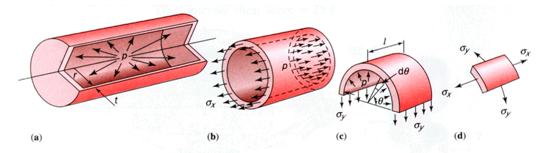Figure 1.3 Stress acting on wall of cylinder.
Consider a thin cylinder of internal radius r, length L and wall thickness t.  The cylinder is under internal pressure p as shown in Figure 2.3.
The major stresses acting on cylinder wall is axial and tangential stresses.
Tangential stress
σy = Pr/t = Pd/2t
Equation 1
Axial stress
σx = Pr/2t = Pd/4t
Equation 2
The axial stress and tangential stress of LPG cylinder are calculated below.
Tangential stress, σy = Pd/2t = (2.5 x 314.4) / (2 x 2.5) = 157.2MPa
Axial stress, σx =Pd/4t = (2 x 314.4) / (4 x 2.5) = 78.6MPa

## 1.3 Methodology.

After proper background study the theoretical values are calculated. The Analysis is done for existing cylinder shape. The model is idealized and only the pressurized part is considered for analysis. Optimization in existing shape are considered for analysis by changing end shapes and overall shape of the cylinder.

## 1.4 Modeling and analysis of pressurized part of cylinder.

### 1.4.1 Analysis using Plane 42 and Plane 82

Plane 42 and Plane 82 are elements having elemental behavior of axisymmetric. So using this element, the problem is solved in axisymmetric approach. For that only cross sectional area is required. In this approach, y axis is the axis of symmetry. Axial stress is the stress along y axis and tangential stress is the stress along z axis. The major difference is that plane 42 is linear and plane 82 is quad elements.
For modeling, proe is the software used. The dimensions such as inner diameter, external height and thickness are considered from Figure 1.2. An acceptable approximation of a 2: 1 Ellipsoidal head is one with a knuckle radius of 0.17D and a spherical radius of 0.90D. As part of idealization only pressurized part of cylinder is made. The welding of lower and upper half of cylinder is not considered of analysis.

For both the elements, as it is approached using axisymmetric concept, modeling and boundary conditions remains the same. A surface is made with required dimension using proe using fill option and is imported as iges. Then area is splited to 5 no. of pieces: 2 caped ends, 2 fillets and one vertical wall. Then for manual meshing, the size is controlled. After mapped messing, boundary conditions are applied. Figure 2.4 shows the loads and boundary conditions applied.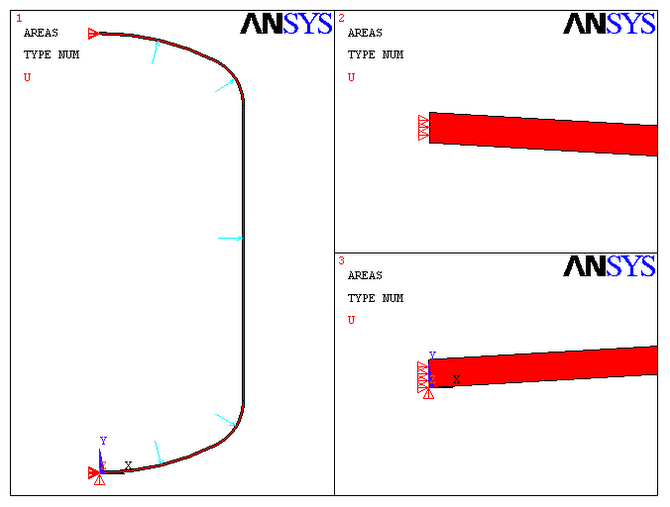Figure 2.4 Loads and boundary conditions applied in Ansys

Figure 2.5 shows the edges that are controlled for mapped meshing.Figure 2.5 Size control for mapped meshing.
Table Two Analysis report with various meshes.
 SET No. NO. OF DIVISIONS PLANE 42 PLANE 82 1 A B C D σy σz σy σz 2 1 50 20 100 149.147 171.628 151.619 171.718 3 1 100 40 200 150.894 171.718 151.604 171.756 4 1 150 50 300 151.083 171.691 151.559 171.732 5 2 150 50 300 151.191 171.706 151.51 171.701 6 2 200 60 400 151.251 171.712 151.454 171.704 7 3 200 60 400 151.326 171.704 151.481 171.699
Table 2.2 shows the no of divisions applied in various lines for size control. Nodal solutions, the axial stress (σy) and tangential stress (σz) for various elements are also tabulated.
In both element, y component of stress shows the axial and z component of stress shows the tangential stress. Our calculated value of axial stress is in range of the analysis result. But the tangential stress (z component) obtained by the analytical method is not matching with the theoretical value.
While using plane 42, we need more no of elements for the convergence of result as the element is linear. If we use plane 82 the convergence is much aquarate with less no of elements. The convergence to 151 on stress in y and 171 on stress in z is reached in set 1 for plane 82 element but to reach that accurate convergence with plane 42 we have to go much heigher no of elements. While analysing 6th and 7th sets, we can identify that both stress values reached to a convergence. But the accuracy of 6th set of plane 82 is same to 7th set of plane 42. The no of elements used in plane 82 is 2/3 of plane 42.
An approximate calculation of no. of elements is shown below.
No. of elements used in 7th set of plane 42= 3 x [(2 x 200)+(2 x 60)+400]=2760

No. of elements used in 6th set of plane 82= 2 x [(2 x 200)+(2 x 60)+400]=1840Figure 2.6 Stress in y and z axis using Plane 42 (Set 7)

Figure 2.5 shows the Stress in y and z axis using Plane 42 element. The axial stress acting is 151.33 and tangential stress is 171.704. The axial stress and tangential stress doesn’t match the theoretical value.Figure 2.7 Stress in y and z axis using Plane 82 (Set 6)
Figure 2.7 shows the Stress in y and z axis using Plane 82 element. The axial stress acting is 151.45 and tangential stress is 171.704. The axial stress and tangential stress doesn’t match the theoretical value. The results plotted in figure 2.6 and 2.7 are nodal solutions.
The actual theoretical value is the stress exerted on vertical wall of cylinder. To plot the exact theoretical value we have to go with path operation. First we have to define a path through the nodes on same line in the vertical wall area. Then map stress on to define path: stress in y as axial and stress in z as hoops (tangential).
If we plot graph of axial and hoops stress along the path, we will get the result matching to the theoretical value. There will not be much variation in no of elements used in Plane 42 and Plane 82.
Figure 2.8 shows the graph of axial and tangential stress exerted on vertical walls of cylinder using Plane 42.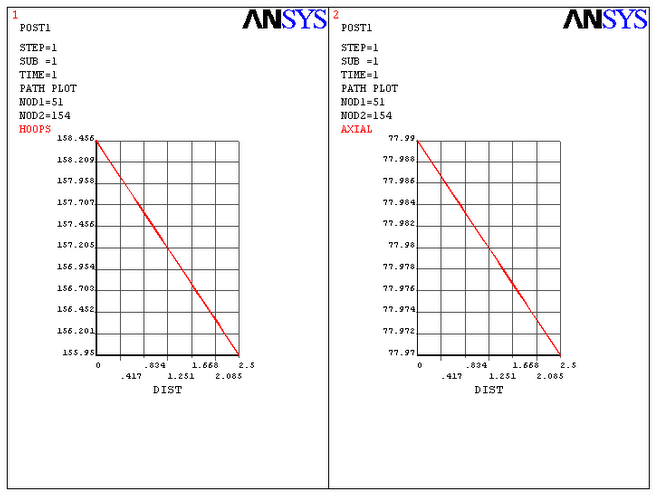Figure 2.8 Axial and hoops stress in vertical wall using Plane 42

Figure 2.9 shows the axial and tangential stress exerted on vertical walls of cylinder using Plane 82.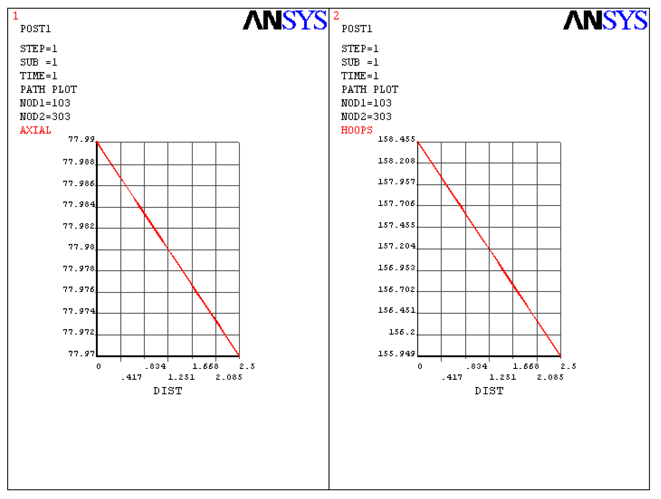Figure 2.9 Axial and hoops stress in vertical wall using Plane 82

### 1.4.2 Similarities and differences

The tangential stress value is converging to 171.704 in both the cases. But the axial stress value is not converged. The major difference is the no of elements used. Path plot gives result that matches with theoretical value. But it is difficult to identify what will be happening at each area especially at capped ends. For easier comparison, nodal solutions of stress in y and z axis are considered throughout the procedures.

## 1.5 Design improvements and optimization

The pressure vessel can be optimized by rethinking the shape of the cylinder. Flat ends and spherical ends are considered for new shapes. Spherical Vessels are considered

### 1.5.1 Analysis of flat end cylinders

In case of flat end cylinder, the stress concentration is high in corner fillets.
Figure 2.8 shows the axial stress in flat end cylinders. In that, the overall stress is in range is in range of 280. But in the areas of fillet the stress rises tremendously. The overall displacement (deformation) is also high.

Figure 2.9 shows the tangential stress in flat end cylinders. In that, the overall stress is in range is in range of 760. But in the areas of fillet, the stress rises tremendously. The overall displacement (deformation) is also high. The capped end is exerted with higher amount of stress. The values may be wrong but the concentration of stress will be accurate. The hoops stress in flat end is much higher compared to other shapes.Figure 2.10 Stress in y axis using Plane 82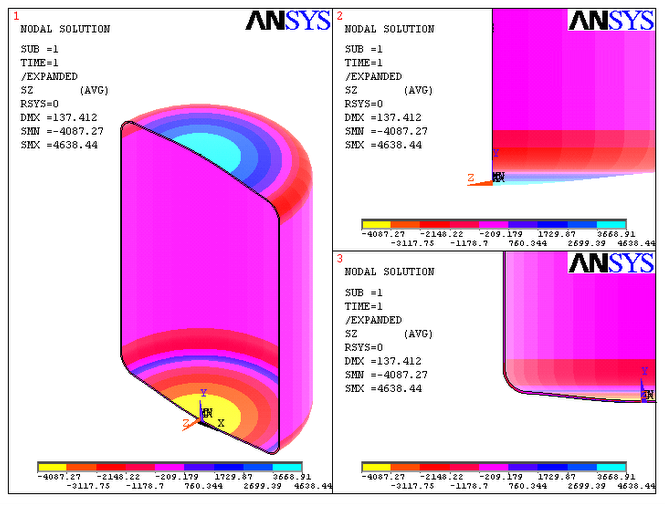Figure 2.11 Stress in z axis using Plane 82

### 1.5.2 Analysis of spherical end cylinders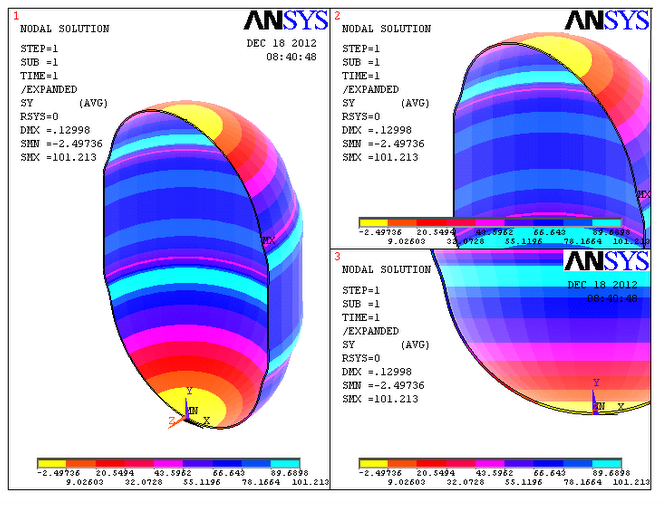Figure 2.12 Stress in y axis using Plane 82Figure 2.13 Stress in z axis using Plane 82
In case of spherical end cylinder, the values of axial and tangential stress are reduced. If we consider the analysis report of curved end type cylinders, the stress concentration is less than that.
Figure 2.10 shows the axial stress in spherical end cylinders. In that, the maximum stress is in range is in range of 101.It is much lower than curved end cylinder. The displacement (deformation) is also low.
Figure 2.11 shows the tangential stress in spherical end cylinders. In that, the maximum stress is in range is in range of 101.It is much lower than curved end cylinder. The displacement (deformation) is also low. The spherical area can withstand much stress. So we can reduce wall thickness only on capped area. The manufacturing of this type is much difficult.

### 1.5.3 Spherical pressure vessel.

The water capacity of 14.2 kg cylinder is 33 L
Volume =4Πr3 /3= 0.33 m3
Inner radius r = 199. 02 mm
Consider a sphere with radius r and wall thickness t filled with gas or fluid with pressure p.
Stresses in all directions are same. Stress exerted on walls σ = p r / 2t
The maximum stress exerted in the case of curved end cylinders are 171 MPa.
Wall thickness t= p r / 2 σ = (2.5x 199.02) / (2 x 171) = 1.46 mm
For analysis, consider
Wall thickness = 1.5 mmFigure 2.14 Stress in x, y & z axis using Plane 82
The stress value is 166.67 and the wall thickness considered is 1.5 mm
So a spherical cylindrical vessel with 200 mm internal radius and wall thickness 1.5 mm can hold a pressure of 2.5 MPa.
The advantage of using spherical pressure vessel is that, the wall thickness is lower compared to other shaped pressure vessel. That reduces the weight of pressure vessel and hence used is ships etc.
The main disadvantage is that it consumes larger floor area compared to other types. It makes transportation difficult. The manufacturing of spherical vessel is much difficult compared to other shapes.

Cylinders ate transported through trucks. The uneven road and during loading and unloading, these experiences various vibrations. Vibrations are nothing else but disturbances which can be ideally considered as certain frequency. These frequencies can be calculated by Modal analysis in ansys.
If the forced frequency matches the natural frequency, the component undergoes failure. Since mass and loads acting will change the frequencies, it is better to calculate the natural frequency of filled cylinder than empty cylinder.
As part of idealization, only pressure vessel area is taken for analysis. Welding, handle and base are avoided.

If we consider empty cylinder, there will not be much disaster.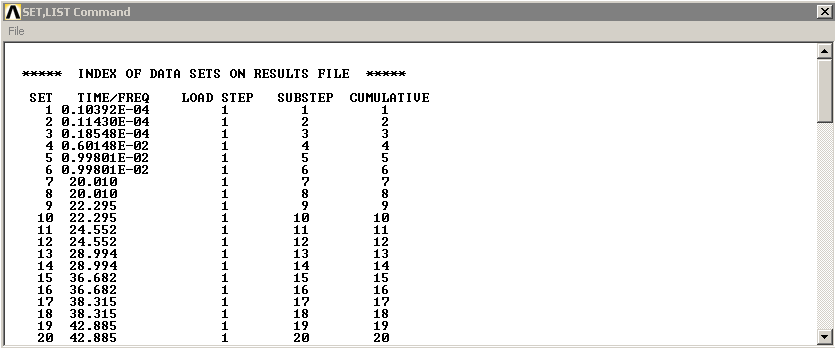Figure 2.15 Frequencies of empty cylinder.
Figure 2.13 shows the first 20 frequencies. The first six values will be their 6 degrees of freedom and 7th value 20.010 can be considered as the natural frequency of empty cylinder. That will be the first frequency that causes catastrophic failure of empty cylinder.

The main concern is in the case of filled cylinders that cause major destruction. So first we perform a static analysis with 2.5MPa pressure and the pre-stress effects are included for modal analysis. For modal analysis we consider 20 mode shapes. We will get many no. of frequencies as we analyze for large no. of mode shapes.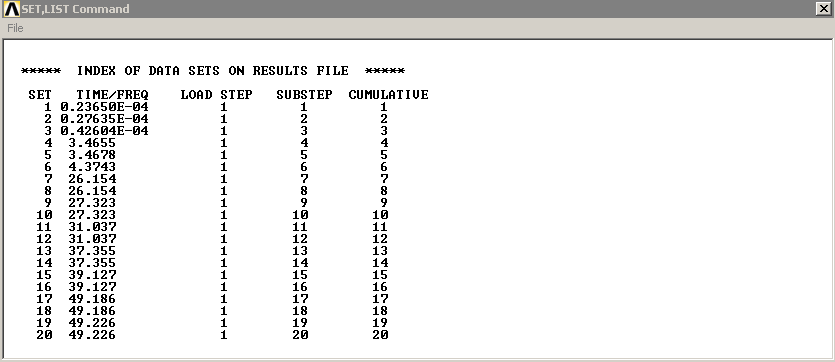Figure 2.16 Frequencies of Filled cylinder.
Figure 2.14 shows the first 20 frequencies of a pressurized cylinder at 2.5MPa. The first six values will be their 6 degrees of freedom and 7th value 26.154 can be considered as the natural frequency of filled cylinder. That will be the first frequency that causes catastrophic failure of filled cylinder.

## 1.7 Conclusion.

From all these analysis done, the shape that can be used is spherical vessels as the wall thickness and weight can be reduced. Due to its difficulty in transportation, spherical end vessels can be used as the cost of cylinder will increase. The frequency of disturbance during loading unloading and transportation will not match with natural frequencies of empty (20.010) as well as filled (26.154) cylinder as these frequencies cause the failure of gas cylinder.

#### References

1. Miss Cheah (December 21, 2011) Vital Knowledge about LPG Gas Cylinder. www.blogspot.inhttp://shuangxingfu.blogspot.in/2011/12/vital-knowledge-about-lpg-gas-cylinders.html (16-12-2012)
2. Laxmikant D. Rangari, P. M. Zode & P.G. Mehar (2012) International Journal of Applied Research in Mechanical Engineering (IJARME) ISSN: 2231 –5950, Volume-2, Issue-1, 2012
3. Technical drawing of  LPG Cylinder from Hindustan petroleum corporation Ltd.http://tenders.hpcl.co.in/tenders/tender_prog/TenderFiles/649/Tender/p1.drawing%2014.2%20kg%20cylinder.pdf
4. International Journal of Applied Research in Mechanical Engineering (IJARME)ISSN: 2231 –5950, Volume-2, Issue-1, 2012